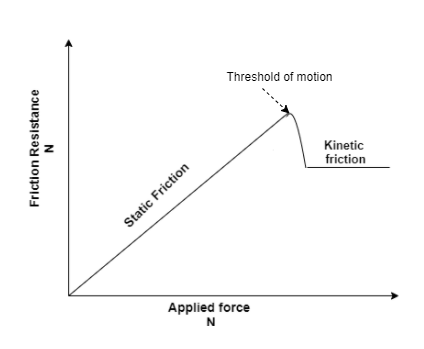# Problem: Which one of the following statements is true?a. Kinetic friction is always greater than the maximum value of static frictionb. Static friction is always equal to kinetic frictionc. Static friction is always equal to µSNd. The maximum value of static friction is µSN

###### FREE Expert Solution

The relationship between static friction and kinetic friction can be shown in the diagram below.90% (329 ratings)###### Problem Details

Which one of the following statements is true?

a. Kinetic friction is always greater than the maximum value of static friction

b. Static friction is always equal to kinetic friction

c. Static friction is always equal to µSN

d. The maximum value of static friction is µSN

Frequently Asked Questions

What scientific concept do you need to know in order to solve this problem?

Our tutors have indicated that to solve this problem you will need to apply the Stacked Blocks concept. You can view video lessons to learn Stacked Blocks. Or if you need more Stacked Blocks practice, you can also practice Stacked Blocks practice problems.